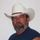cancel
Showing results for
Did you mean:Level 3

# Should the Balance Summary Sheet update in the Inventory Asset-1 to make both quantity and cost 0 when an invoice is generated for an item sold?

1 Comment 1Level 15

## Should the Balance Summary Sheet update in the Inventory Asset-1 to make both quantity and cost 0 when an invoice is generated for an item sold?

maybe

QB uses average cost for inventory valuation, FIFO which is what QBO uses is just average cost per purchase still the item cost is average cost.

If you buy 5ea for \$25, then average cost is easy, \$25 / 5 = \$5 each   So yes you sell one and the average cost of \$5 posts to COGS

Intuit programmers dropped the ball though.

buy 3ea for \$10 and the average cost is \$3.3333333

but costs is rounded to two digits, so when you sell one, 3.33 is posted to COGS

when only one is in stock, the average cost is .03333

sell it and 3.33 is posted,

3 * 3.333 = 9.999

10 original cost less 9.999 = .001 or some other amount due to rounding left over

over time that can add up, and there is no way to know what purchase(s) is causing the issue, there is no way to adjust item cost either.

## Need to get in touch?

Sitemap